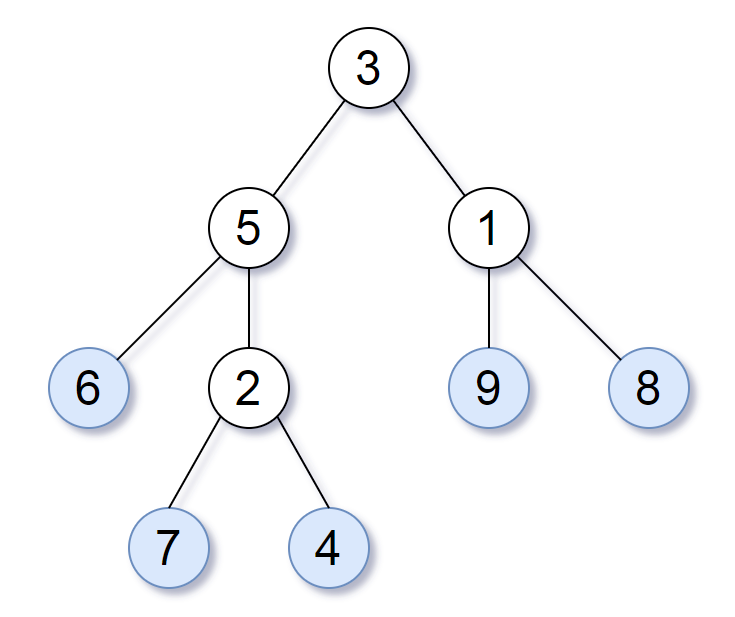# Leaf-Similar trees

Title: Leaf-Similar TreesSource: leetcode.com

Consider all the leaves of a binary tree. From left to right order, the values of those leaves form a leaf value sequence.Leaves in a tree

For example, in the given tree above, the leaf value sequence is (6, 7, 4, 9, 8).

Two binary trees are considered leaf-similar if their leaf value sequence is the same.

Return true if and only if the two given trees with head nodes root1 and root2 are leaf-similar.

Note:

Both of the given trees will have between 1 and 100 nodes.

### Java solution

Leaf-Similar trees
Rate this post